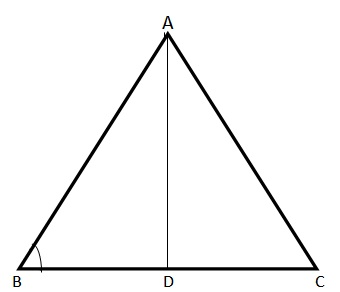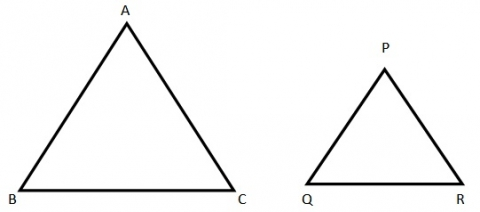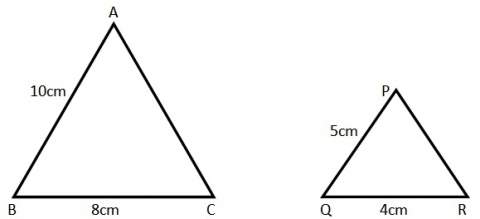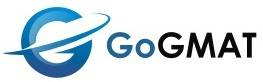# Triangle Types and Similar Triangles

A triangle is a closed figure formed by three straight lines provided the sum of angles formed between the lines is 180 degrees. There are six types of triangles

• Isosceles Triangle: A triangle with two sides of equal length and corresponding angles of equal measure.

Example:
Triangle with the angles 30°, 30° and 120°.

• Equilateral Triangle: A triangle that has all three sides of equal length and all three angles of equal measure. As the sum of the three angles must equal 180°, each angle equals 60° in an equilateral triangle.

• Scalene Triangle: If all the three sides of the triangle are of different size, then it is a scalene triangle.

Example:
A triangle with sides 3cm, 4cm and 5cm is scalene.

• Right Triangle: A triangle that has one 90° angle. •    Acute Triangle: A triangle with all angles less than 90°.

Example:
A triangle formed with angles 50°, 60° and 70° is an acute triangle

Obtuse Triangle: An obtuse triangle is a triangle with one angle greater than 90° o

Example: Triangle with angles 30°, 30° and 120°.

Commonly used Terms

Altitude:
The perpendicular distance from the base to the opposite vertex.

Base:
We can take any side of the triangle as the base, for the purposes of further calculation.Vertex: Angles of triangles are called vertices.

Any one of AB, BC or AC could be taken as the base. In this case, AD is the Altitude, and BC is the base. Angle A, Angle B and Angle C are the vertices of the triangle.

Similar triangles

Similar triangles have the same shape, but not necessarily the same size.The similarity of triangles is based on one of the following three scenarios:

SSS Similarity: If each pair of corresponding sides has the same ratio, then the triangles are said to be similar.

So in the above figure, If AB/PQ = BC/QR = AC/PR, then the triangles are said to be similar, and written as ABC ~ PQR

AAA Similarity: If the corresponding angles of the given triangles are of the same measurement, then the triangles are said to be of AAA similarity.

So in the above figure if Angle A = Angle P, Angle B = Angle Q and Angle C = Angle R, then the triangles are similar.

SAS Similarity:
If two corresponding sides of the triangles are of the same ratio and the angles formed by these sides are of the same measurement, then the triangles are said to be of SAS similarity.

In the above figure, if Angle A = Angle P and AB/PQ = AC/PR, then the triangles are similar.

GMAT problems on Triangles

1) Given that two triangles ABC and PQR are similar. AB = 10cm, BC = 8cm, Angle A=Angle B=60°. PQ = 5cm, QR=4cm. Find PR and ACSolution: One needs to apply two concepts to get the values of PR and AC Given that both the triangles are similar

By following the SSS property, AB/AC = PQ/PR.

So 10cm/8cm = 5cm/PR

10/8 = 5/PR

5/4 = 5/PR

So PR = 4

Therefore PR = 4cm

Now we can find AC in two ways:

Method1:
Given the data Angle A = Angle B = 60°. The triangle is isosceles; therefore the sides opposite the equal angles should also be equal. Hence BC = AC = 8cm

Method 2: We got PR=4cm. Again applying the rule for similar triangles AC/BC = PR/QR

AC/8cm = 4cm/4cm

AC/8 = 4/4

AC/ 8 = 1

AC = 1*8

AC = 8

Therefore AC = 8cm

Important points to remember

• The sum of any two sides of a triangle is always greater than the third side

• If we have the data given for two sides of a triangle, the length of the third side must lie between the difference and sum of the two given sides.

• The smallest side of the triangle will have the smallest angle opposite it
. Similarly, the longest side of the triangle will have the largest angle opposite it.

Author :GoGMAT, founded in 2009, is an adaptive GMAT preparation platform developed by the best instructors in the industry (with 740+ GMAT scores and strong teaching experience). GoGMAT team consists of high profile members, successful entrepreneurs, experts in educational field, top-notch business school alumni with successful track records as investment bankers and management consultants. Our GMAT experts, university professors and successful entrepreneurs, analyzed GMAT test algorithms for almost 12 months.  We used their knowledge and skills as the basis to create one of the most efficient solutions in the world for GMAT preparation.

Disclaimer: The articles provided by GoGMAT are for informational purposes only and cannot be copied or redistributed without the written permission by GoGMAT. F1GMAT publishes guest posts by authors from all over the world. We do not accept any responsibility or liability for the information contained in the article or claims of copyright violation. However, we take immediate action when any copyright violations are reported. Report copyright violation1. Complete GMAT RC Questions in less than 1 minute and 50 seconds
3. Take Notes Effectively
4. Collect and Interpret Facts
5. Speed up Summary Creation
6. Remember Information
7. Question the Author

12. Learn to Answer GMAT organization of passage Question
13. Learn to identify the style/tone or attitude of the author

Mastering GMAT Critical ReasoningAfter you read F1GMAT’s Mastering GMAT Critical Reasoning Guide, you will learn:

How to overcome flawed thinking in GMAT Critical Reasoning?

How to spot Inconsistencies in Arguments

How to eliminate out of scope answer choices using Necessary and Sufficient Conditions

How to Paraphrase GMAT Critical Reasoning Question

How to Answer Assumption Question Type

How to Answer Conclusion Question Type

How to Answer Inference Question Type

How to Answer Strengthen Question Type

How to Answer Weaken Question Type

How to Answer bold-faced and Summary Question Types

How to Answer Parallel Reasoning Questions

How to Answer the Fill in the Blanks Question

Get F1GMAT's Newsletters (Best in the Industry)

• Ranking Analysis
• Post-MBA Salary Trends
• Post-MBA Job Function & Industry Analysis
• Post-MBA City Review
• MBA Application Essay Tips
• School Specific Essay Tips
• GMAT Preparation Tips